Courses

# Mechanism - MCQ Test 2

## 30 Questions MCQ Test RRB JE for Mechanical Engineering | Mechanism - MCQ Test 2

Description
This mock test of Mechanism - MCQ Test 2 for Mechanical Engineering helps you for every Mechanical Engineering entrance exam. This contains 30 Multiple Choice Questions for Mechanical Engineering Mechanism - MCQ Test 2 (mcq) to study with solutions a complete question bank. The solved questions answers in this Mechanism - MCQ Test 2 quiz give you a good mix of easy questions and tough questions. Mechanical Engineering students definitely take this Mechanism - MCQ Test 2 exercise for a better result in the exam. You can find other Mechanism - MCQ Test 2 extra questions, long questions & short questions for Mechanical Engineering on EduRev as well by searching above.
QUESTION: 1

### The figure below shows a planar mechanism with single degree of freedom. The instant centre 24 for the given configuration is located at a position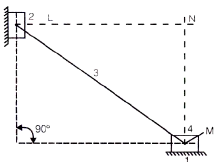[GATE-2004]

Solution: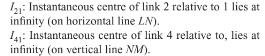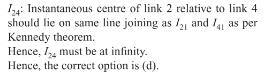QUESTION: 2

### In the figure shown, the relative velocity of link 1 with respect to link 2 is 12 m/sec. Link 2 rotates at a constant speed of 120 rpm. The magnitude of Carioles component of acceleration of link 1 is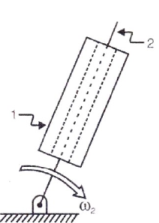Solution:

Velocity of link 1 with respect to 2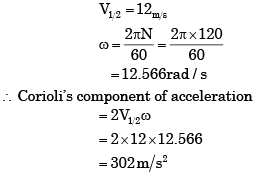QUESTION: 3

### The coupling used to connect two shafts with large angular misalignment is      [GATE-2002]

Solution:
QUESTION: 4

For the audio cassette mechanism shown in Figure given below where is the instantaneous centre of rotation (point) of the two spools?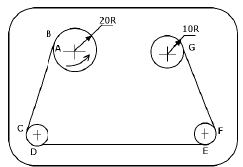[GATE-1999]

Solution:
QUESTION: 5

Match List I with List II and select the correct answer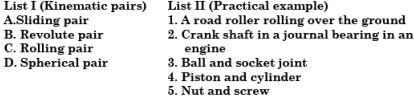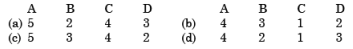[IES-2002]

Solution:

Sliding pair→ piston and cylinder
Revolute pair → Crank shaft in a journal bearing in an engine
Rolling →A road roller rolling over the ground
Spherical pair→ Ball and socket joint

QUESTION: 6

The Carioles component of acceleration is present

[GATE-2002]

Solution:

When a point on one link is sliding along another rotating link, such as in quick return motion mechanism, then the coriolis component of the acceleration must be calculated. Quick return motion mechanism is used in shaping machines, slotting machines and in rotary internal combustion engines.

QUESTION: 7

Consider the following statements:

1. The degree of freedom for lower kinematic pairs is always equal to one.
2. A ball-and-socket joint has 3 degrees of freedom and is a higher kinematic pair
3.Oldham's coupling mechanism has two prismatic pairs and two revolute pairs.

Which of the statements given above is/are correct?

[IES-2005]

Solution:
QUESTION: 8

A round bar A passes   through the cylindrical hole in B as shown in the given figure. Which one of the following statements is correct in this regard?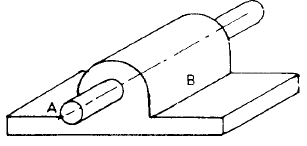[IES-1995]

Solution:

When two elements or links are connected in such a way that their relative motion is constrained they form a kinematic pair. The relative motion of a kinematic pair may be completely, incompletely or successfully constrained

QUESTION: 9

Which of the following are examples of forced closed kinematic pairs?

1. Cam and roller mechanism
2. Door closing mechanism
3. Slider-crank mechanism
4. Automotive clutch operating mechanism

Select the correct answer using the codes given below:

[IES-2003]

Solution:

Forced closed kinematic pairs – If the contact between the two pairs is maintained by application of force.

Door closing mechanism : It forces the door to be closed when opened.

QUESTION: 10

Consider the following statements

1. A round bar in a round hole form a turning pair.
2. A square bar in a square hole forms a sliding pair.
3. A vertical shaft in a footstep bearing forms a successful constraint.
Of these statements

[IES-2000]

Solution:

If round bar is placed in a round hole it will have both turning and as well as sliding pair also.

QUESTION: 11

Assertion (A): Hydraulic fluid is one form a link.

Reason (R): A link need not necessarily be a rigid body but it must be a resistant body.

[IES-1996]

Solution:
QUESTION: 12

Assertion (A): The elements of higher pairs must be force closed.

Reason (R): This is required in order to provide completely constrained motion.

[IES-1995]

Solution:

Elements of higher pairs must be force closed to provide completely constrained motion.

QUESTION: 13

Assertion (A): When a link has pure translation, the resultant force must pass through the centre of gravity.

Reason (R): The direction of the resultant force would be in the direction of acceleration of the body.

[IES-1994]

Solution:
QUESTION: 14

Consider the following pairs of parts:

1. Pair of gear in mesh
2. Belt and pulley
3. Cylinder and piston
4. Cam and follower Among these, the higher pairs are

[IES-2000]

Solution:
QUESTION: 15

Consider the following pairs of parts:

1. Pair of gear in mesh
2. Belt and pulley
3. Cylinder and piston
4. Cam and follower Among these, the higher pairs are

[IES-2000]

Solution:
QUESTION: 16

In a Kinematic chain, a quaternary joint is equivalent to:

[IES-2005]

Solution:

When ‘l’ number of links are joined at the same connection, the joint is equivalent to (l - 1) binary joints.

QUESTION: 17

Consider the following statements:

1. Lower pairs are more resistant than the higher pairs in a plane mechanism.
2. In a 4-bar mechanism (with 4 turning pairs), when the link opposite to the shortest link is fixed, a double rocker mechanism results.

Which of the statements given above is/are correct?

[IES-2006]

Solution:
QUESTION: 18

Which of the following are examples of a kinematic chain?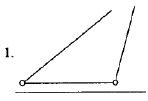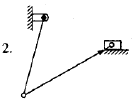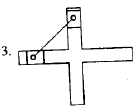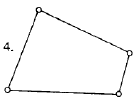Select the correct answer using the codes given below:

[IES-1998]

Solution:
QUESTION: 19

Match List-I with List-II and select the correct answer using the codes given below the lists: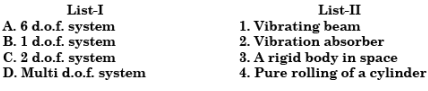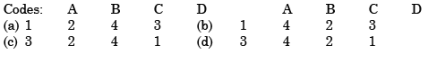Solution:

A rigid body in space has 6 degrees of freedom. A vibrating absorber has two degrees of freedom.

QUESTION: 20

The kinematic chain shown in the above figure is a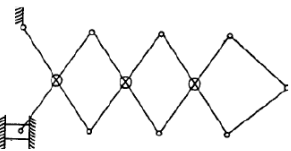[IES-2000]

Solution:
QUESTION: 21

Assertion (A): The elements of higher pairs must be force closed.

Reason (R): This is required in order to provide completely constrained motion.

[IES-1995]

Solution:

Elements of higher pairs must be force closed to provide completely constrained motion.

QUESTION: 22

The two-link system, shown in the given figure, is constrained to move with planar motion. It possesses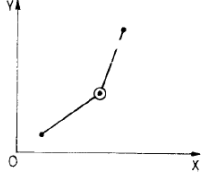Solution:

Two link system shown in the above figure has 2 degrees of freedom.

QUESTION: 23

Assertion (A): When a link has pure translation, the resultant force must pass through the centre of gravity.

Reason (R): The direction of the resultant force would be in the direction of acceleration of the body.

[IES-1994]

Solution:
QUESTION: 24

A linkage is shown below in the figure in which links ABC and DEF are ternary Jinks whereas AF, BE and CD are binary links.

The degrees of freedom of the linkage when link ABC is fixed are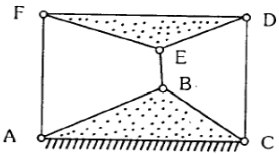[IES-2002]

Solution:
QUESTION: 25

When supported on three points, out of the 12 degrees of freedom the number of degrees of reedom arrested in a body is

[IES-1993]

Solution:

When supported on three points, following six degrees of freedom are arrested (two line movements along y-axis, two rotational movements each along xaxis and z-axis.)

QUESTION: 26

Inversion of a mechanism is

[IES-1992]

Solution:
QUESTION: 27

f = 3 (n - 1) - 2j. In the Grubler's equation for planar mechanisms given, j is the

[IES-2003]

Solution:

In general a mechanism with n number of links connected by j number of binary joints or lower pairs since it is single degree of freedom.

QUESTION: 28

Which one of the following conversions is used by a lawn-sprinkler which is a four bar mechanisms?

[IES-2004]

Solution:

Generally it will rotate downwards and convert this motion to give oscillations motion as output.

QUESTION: 29

The centre of gravity of the coupler link in a 4-bar mechanism would experience

[IES-1996]

Solution:

The centre of gravity of the couples link in a 4-bar mechanism would experience both linear and angular acceleration.

QUESTION: 30

A four-bar chain has

[IES-2000]

Solution: